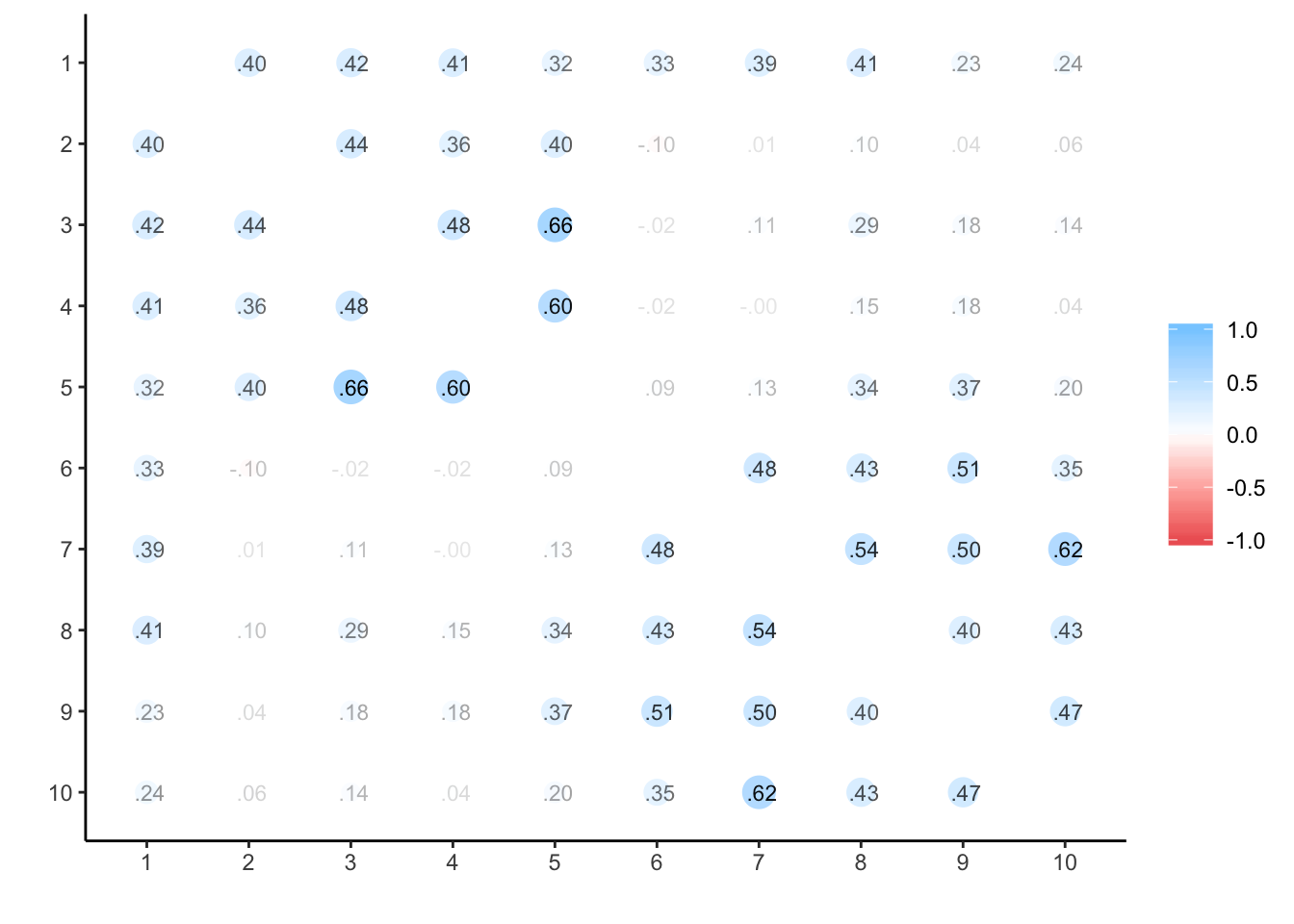# Re-analysis of OCMATE data to get reliability of change

## Pre-process data

# Load packages
library(tidyr)
library(dplyr)
##
## Attaching package: 'dplyr'
## The following objects are masked from 'package:stats':
##
##     filter, lag
## The following objects are masked from 'package:base':
##
##     intersect, setdiff, setequal, union
library(ggplot2)
library(lme4)
## Loading required package: Matrix
##
## Attaching package: 'Matrix'
## The following object is masked from 'package:tidyr':
##
##     expand
library(lmerTest)
##
## Attaching package: 'lmerTest'
## The following object is masked from 'package:lme4':
##
##     lmer
## The following object is masked from 'package:stats':
##
##     step
library(knitr)

options(scipen=999)
knitr::opts_chunk$set(cache=TRUE) # calculate standard errors se <- function(x, na.rm = FALSE) { if (na.rm) { the.SE <- sqrt(var(x,na.rm=TRUE)/length(na.omit(x))) } else { the.SE <- sqrt(var(x,na.rm=FALSE)/length(x)) } return(the.SE) } # short summaries for lmerTest mySummary<-function(lmer_summary) { coefTable <- lmer_summary$coefficients %>%
round(3) %>%
as.data.frame() %>%
rownames_to_column()

if (ncol(coefTable)>5) {
coefTable <- coefTable %>%
mutate(
sig = ifelse(.<.001, " * * * ",
ifelse(.<.01,   " *  * ",
ifelse(.<.05,   " * ",
ifelse(.<.10,   "+", "")))))
}

return(list(lmer_summary$ngrps, kable(coefTable))) } ### Load data Data entered from all white, heterosexual women not using any form of hormonal contraceptives Each row is all data from a single session (i.e. oc_id:date) • “oc_id” = ID of the subject • “block” = testing block (1, 2 or 3) • “block_N” = how many testing sessions completed in that block • “age” = age (in years) of subject on day of testing • “ethnicity” = ethnic group of subject (all white) • “sexpref” = sexual preference of subject (all heterosexual) • “date” = date of testing session • “partner” = does subject currently have a romantic partner? (0 = no partner, 1 = yes partner) • “block_partner” = all unique partner column values in the block (0 if never had partner, 1 if always had partner, otherwised mixed: e.g., 1,0) • “behavior_soi” = behavior subscale of SOI-R • “attitude_soi” = attitude subscale of SOI-R • “desire_soi” = desire subscale of SOI-R • “current_sexdrive”= current sex drive score • “solitary_SDI” = solitary desire subscale of SDI-2 • “dyadic_SDI” = dyadic desire subscale of SDI-2 • “total_SDI” = total score on SDI-2 • “progesterone” = salivary progesterone for that session • “estradiol” = salivary estradiol for that session • “testosterone” = salivary testosterone for that session • “cortisol” = salivary cortisol for that session data_start <- read.csv("OCMATE_sexdrive_anon.csv", stringsAsFactors = F)  #### Re-code variables • partner.e = partnership status (no = -0.5, partner = +0.5, mixed = NA) # wide to long format # effect code partnership status (no = -0.5, partner = 0.5) data_all <- data_start %>% gather(question, score, behavior_soi:total_SDI) %>% mutate( partner.e = ifelse(block_partner==0, -0.5, ifelse(block_partner==1, 0.5, NA)), prog = progesterone, estr = estradiol, test = testosterone, cort = cortisol ) ### Age # mean age at start of testing data_all %>% group_by(oc_id) %>% summarise( min_age = min(age, na.rm = TRUE) ) %>% ungroup() %>% group_by() %>% summarise( n = n(), mean_age = mean(min_age, na.rm = TRUE), sd_age = sd(min_age, na.rm = TRUE) ) %>% t() ## [,1] ## n 375 ## mean_age Inf ## sd_age Inf ### Descriptive Stats for Questionaires data_all %>% group_by(question) %>% summarise( N_valid = sum(!is.na(score)), N_missing = sum(is.na(score)), mean_score = mean(score, na.rm = TRUE), sd_score = sd(score, na.rm = TRUE) ) ## # A tibble: 7 x 5 ## question N_valid N_missing mean_score sd_score ## <chr> <int> <int> <dbl> <dbl> ## 1 attitude_soi 2189 87 9.22 3.50 ## 2 behavior_soi 2140 136 5.74 2.67 ## 3 current_sexdrive 2145 131 3.77 1.56 ## 4 desire_soi 2168 108 8.06 2.96 ## 5 dyadic_SDI 2098 178 35.5 11.9 ## 6 solitary_SDI 2099 177 8.63 6.46 ## 7 total_SDI 2051 225 44.1 15.7 ### The number of sessions completed per woman data_all %>% group_by(oc_id) %>% summarise( sessions = n_distinct(date) ) %>% group_by(sessions) %>% summarise( n = n() ) %>% spread(sessions, n) %>% t() ## [,1] ## 1 15 ## 2 9 ## 3 5 ## 4 9 ## 5 233 ## 6 1 ## 7 1 ## 8 2 ## 9 2 ## 10 98 ### Exclude observations with missing estr, prog and test ## Exclude observations with EPT missing hormone values sub_hormones_no_EPT <- data_all %>% filter( !is.na(prog) | !is.na(estr) | !is.na(test) ) ### Exclude subjects with only a single session in a block This is necessary because you can’t calculate subject-centered means with only one data point. check_single_session <- sub_hormones_no_EPT %>% group_by(oc_id, block) %>% summarise(sessions = n_distinct(date)) %>% ungroup() %>% filter(sessions == 1) sub_hormones_multisession <- sub_hormones_no_EPT %>% anti_join(check_single_session, by=c('oc_id', 'block')) ### Remove outlier hormone values Remove below bottom sensitivity thresholds for assays (progesterone < 5, estrogen < 0.1), and remove outlier values (+/- 3SD from the mean) sub_hormones_no_outliers <- sub_hormones_multisession %>% mutate( prog = ifelse(prog >= 5, prog, NA), estr = ifelse(estr >= 0.1, estr, NA), prog = if_else(prog>mean(prog, na.rm=TRUE) + 3*sd(prog, na.rm=TRUE) | prog<mean(prog, na.rm=TRUE) - 3*sd(prog, na.rm=TRUE), NA_real_, prog), estr = ifelse(estr>mean(estr, na.rm=TRUE) + 3*sd(estr, na.rm=TRUE) | estr<mean(estr, na.rm=TRUE) - 3*sd(estr, na.rm=TRUE), NA, estr), test = ifelse(test>mean(test, na.rm=TRUE) + 3*sd(test, na.rm=TRUE) | test<mean(test, na.rm=TRUE) - 3*sd(test, na.rm=TRUE), NA, test), cort = ifelse(cort>mean(cort, na.rm=TRUE) + 3*sd(cort, na.rm=TRUE) | cort<mean(cort, na.rm=TRUE) - 3*sd(cort, na.rm=TRUE), NA, cort) ) qplot(sub_hormones_no_outliers$prog)
## stat_bin() using bins = 30. Pick better value with binwidth.
## Warning: Removed 287 rows containing non-finite values (stat_bin).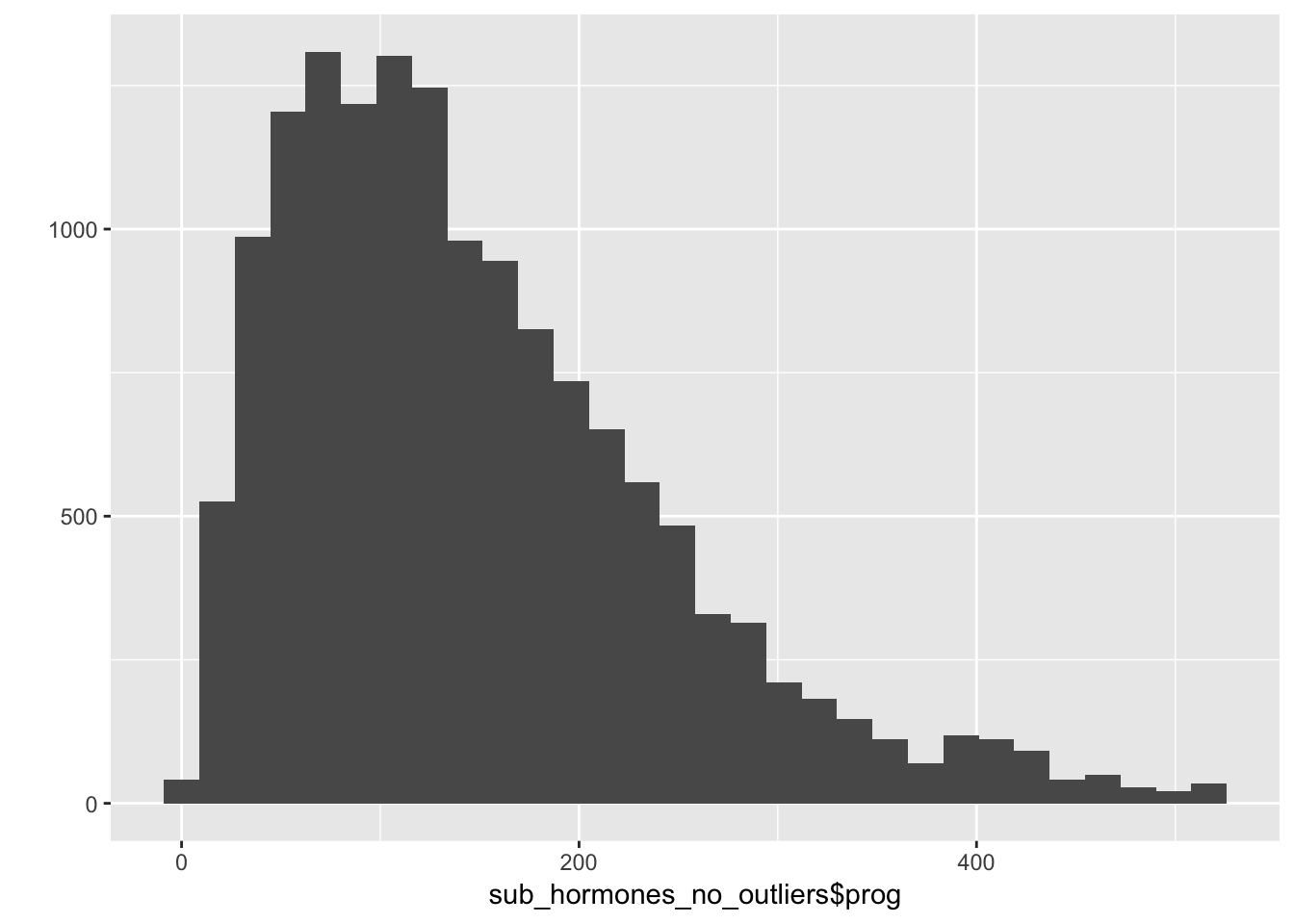# how many included?
check_hormone_exclusions <- sub_hormones_no_outliers %>%
group_by(oc_id, date) %>%
summarise(
e = is.na(mean(estr)),
p = is.na(mean(prog)),
t = is.na(mean(test)),
c = is.na(mean(cort))
) %>%
ungroup() %>%
select(e:c) %>%
gather('hormone','na', e:c) %>%
group_by(hormone) %>%
summarise(
'valid' = n() - sum(na),
'excluded' = sum(na)
) %>%
arrange(hormone)

kable(check_hormone_exclusions)
hormone valid excluded
c 2155 11
e 2146 20
p 2125 41
t 2143 23
  check_hormone_exclusions %>%
group_by() %>%
summarise(
total_hormone_samples_valid = sum(valid),
total_hormone_samples_excluded = sum(excluded)
) %>% gather("stat", "value", 1:length(.))
## # A tibble: 2 x 2
##   stat                           value
##   <chr>                          <int>
## 1 total_hormone_samples_valid     8569
## 2 total_hormone_samples_excluded    95

### Subject-mean-centre hormones

Divide results by a constant to put all hormones on ~ -0.5 to +0.5 scale

# subject-mean-centre hormones
# and divide by a constant to put all hormones on ~ -0.5 to +0.5 scale

data_hormones <- sub_hormones_no_outliers %>%
group_by(oc_id) %>%
mutate(prog.s = (prog-mean(prog, na.rm=TRUE))/400,
estr.s = (estr-mean(estr, na.rm=TRUE))/5,
test.s = (test-mean(test, na.rm=TRUE))/100,
cort.s = (cort-mean(cort, na.rm=TRUE))/0.5,
ep_ratio.s = ((estr/prog)-mean(estr/prog, na.rm=TRUE))/0.075) %>%
ungroup() %>%
as.data.frame()

data_hormones %>%
group_by(oc_id, date, prog.s, estr.s, test.s, cort.s, ep_ratio.s) %>%
summarise(n = n()) %>%
ungroup() %>%
gather("hormone", "value", prog.s:ep_ratio.s) %>%
ggplot(aes(value, colour=hormone)) +
geom_density(alpha=.5) +
scale_x_continuous(limits = c(-1,1))
## Warning: Removed 200 rows containing non-finite values (stat_density).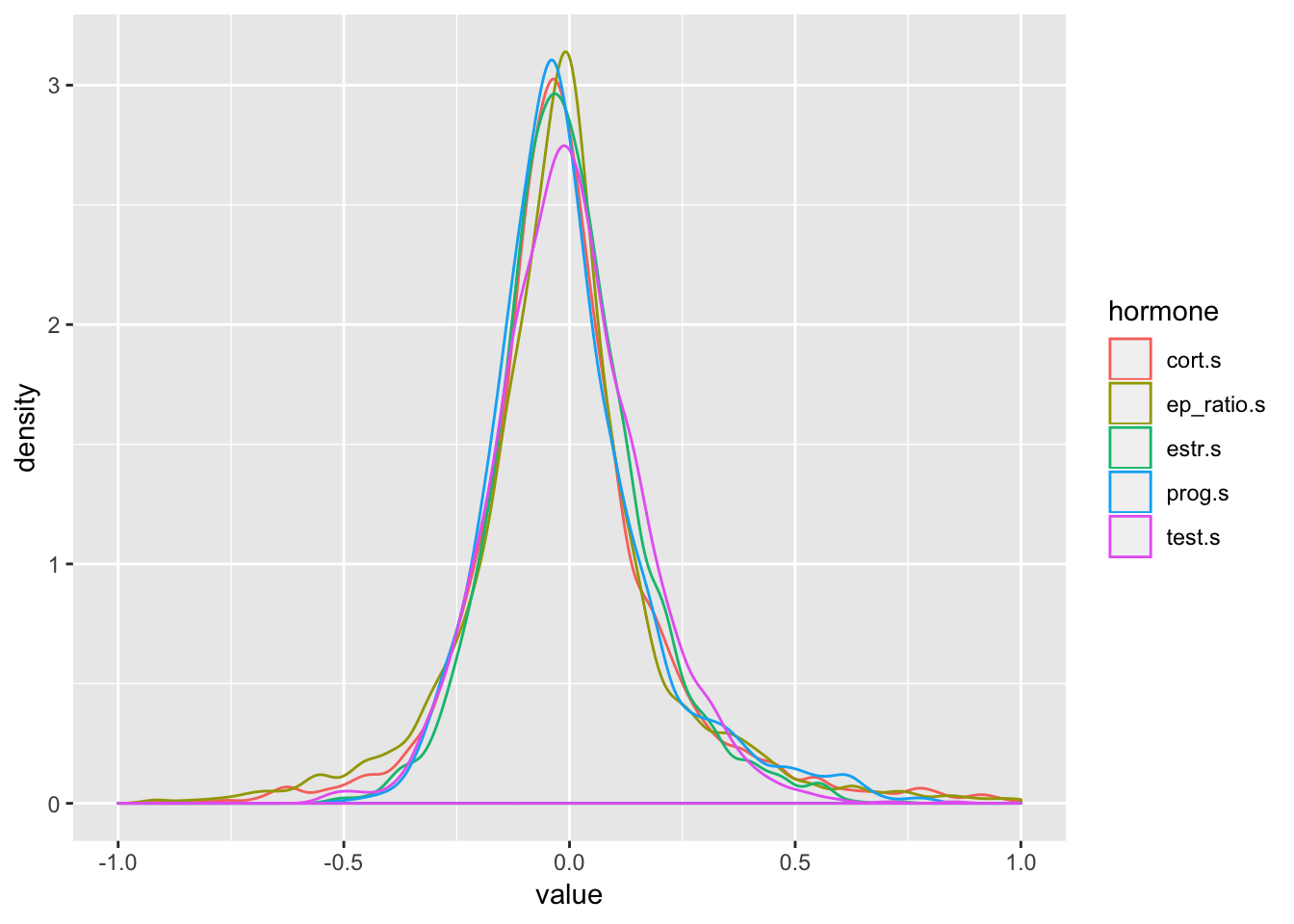### Mean Hormone Levels

data_hormones %>%
group_by(oc_id, date, prog, estr, test, cort) %>%
summarise(n = n()) %>%
ungroup() %>%
group_by() %>%
summarise(
mean_prog = mean(prog, na.rm = TRUE),
sd_prog =   sd(prog, na.rm = TRUE),
se_prog =   se(prog, na.rm = TRUE),
mean_estr = mean(estr, na.rm = TRUE),
sd_estr =   sd(estr, na.rm = TRUE),
se_estr =   se(estr, na.rm = TRUE),
mean_test = mean(test, na.rm = TRUE),
sd_test =   sd(test, na.rm = TRUE),
se_test =   se(test, na.rm = TRUE),
mean_cort = mean(cort, na.rm = TRUE),
sd_cort =   sd(cort, na.rm = TRUE),
se_cort =   se(cort, na.rm = TRUE)
) %>% gather("stat", "value", 1:length(.)) %>%
mutate(value = round(value, 4)) %>%
separate(stat, c("stat", "hormone")) %>%
spread(stat, value)
## # A tibble: 4 x 4
##   hormone    mean     sd     se
##   <chr>     <dbl>  <dbl>  <dbl>
## 1 cort      0.229  0.164 0.0035
## 2 estr      3.30   1.27  0.0275
## 3 prog    149.    96.2   2.09
## 4 test     87.6   27.2   0.587

### Partnership

Exclude blocks with partner inconsistently reported and women who change partnership status between blocks, only for analyses considering partnership status

data_hormones_partner <- data_hormones %>%
filter(block_partner == "0" | block_partner =="1") %>%
group_by(oc_id) %>%
mutate(pchange = mean(partner.e)) %>%
ungroup() %>%
filter(pchange %in% c(-.5, .5)) %>%
select(-pchange)

## Multilevel Reliability / Generalizability Analyses

library(psych)
data_wide = data_hormones %>% select(oc_id, date, block, estr, prog, test, cort) %>% unique()
data_wide <- data_wide %>% group_by(oc_id) %>%
arrange(oc_id, date) %>%
mutate(timepoint = row_number()) %>%
ungroup() %>%  as.data.frame()

multilevel.reliability(data_wide %>% select(oc_id, date, estr), "oc_id", "date", 3, aov = F)
## Warning in cov2cor(C): diag(.) had 0 or NA entries; non-finite result is
## doubtful
##
## Multilevel Generalizability analysis
## Call: multilevel.reliability(x = data_wide %>% select(oc_id, date,
##     estr), grp = "oc_id", Time = "date", items = 3, aov = F)
##
## The data had  352  observations taken over  306  time intervals for  1 items.
##
##  Alternative estimates of reliability based upon Generalizability theory
##
## RkRn =  1 Generalizability of between person differences averaged over time (time nested within people)
## Rcn  =  0.83 Generalizability of within person variations averaged over items  (time nested within people)
##  The nested components of variance estimated from lme are:
##          Variance Percent
## id           0.92   0.548
## id(time)     0.63   0.377
## residual     0.13   0.075
## total        1.68   1.000
##
## To see the ANOVA and alpha by subject, use the short = FALSE option.
##  To see the summaries of the ICCs by subject and time, use all=TRUE
##  To see specific objects select from the following list:
##  ANOVA s.lmer s.lme alpha summary.by.person summary.by.time ICC.by.person ICC.by.time lmer long Call
data_wide %>%
select(oc_id, timepoint, estr) %>%
select(-oc_id) %>%
cor(use='na.or.complete') %>%
corrr::rplot(print_cor = T)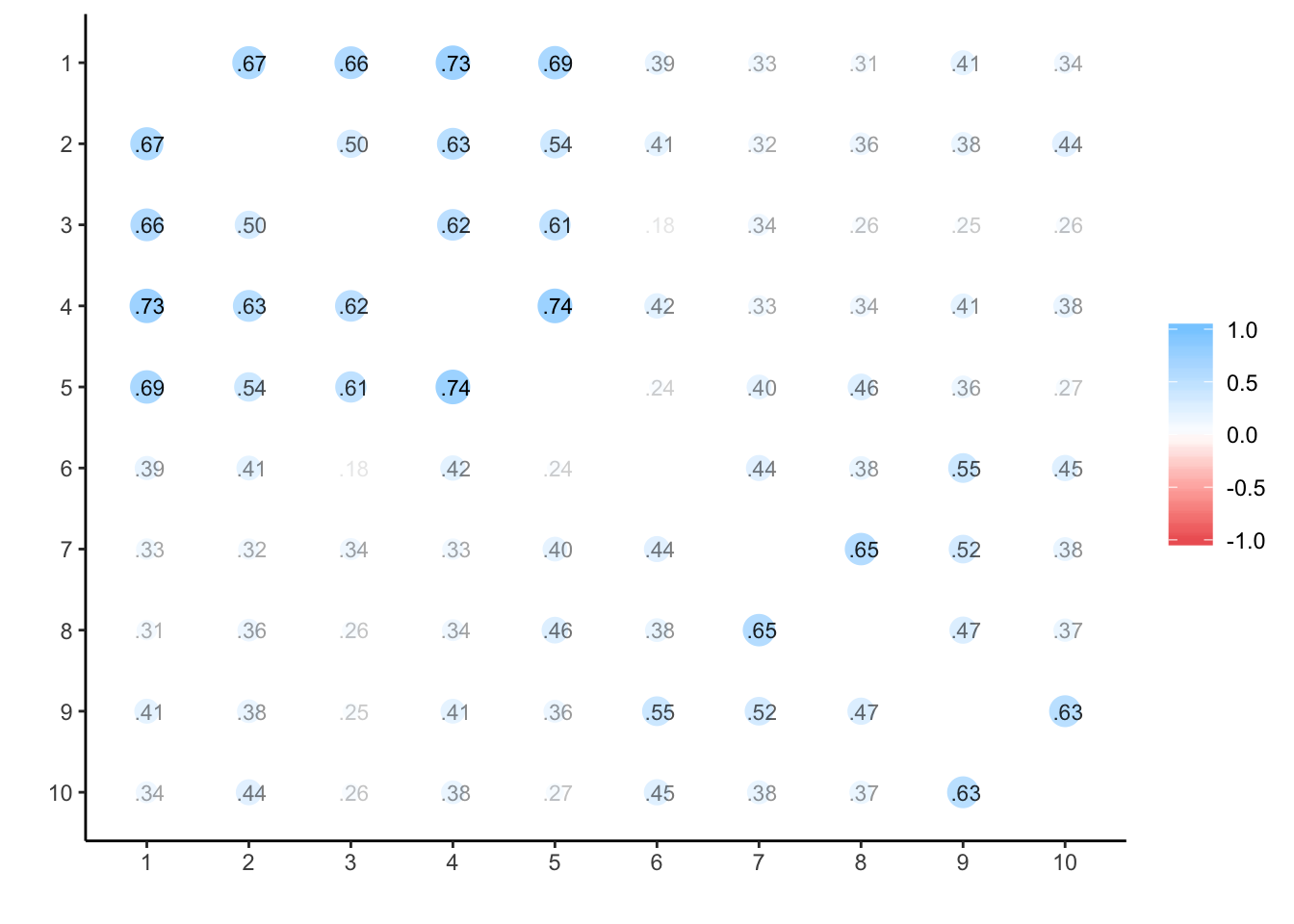### Progesterone

multilevel.reliability(data_wide %>% select(oc_id, date, prog), "oc_id", "date", 3, aov = F)
##
## Multilevel Generalizability analysis
## Call: multilevel.reliability(x = data_wide %>% select(oc_id, date,
##     prog), grp = "oc_id", Time = "date", items = 3, aov = F)
##
## The data had  352  observations taken over  306  time intervals for  1 items.
##
##  Alternative estimates of reliability based upon Generalizability theory
##
## RkRn =  0.99 Generalizability of between person differences averaged over time (time nested within people)
## Rcn  =  0.86 Generalizability of within person variations averaged over items  (time nested within people)
##  The nested components of variance estimated from lme are:
##          Variance Percent
## id           3014   0.324
## id(time)     5392   0.579
## residual      899   0.097
## total        9305   1.000
##
## To see the ANOVA and alpha by subject, use the short = FALSE option.
##  To see the summaries of the ICCs by subject and time, use all=TRUE
##  To see specific objects select from the following list:
##  ANOVA s.lmer s.lme alpha summary.by.person summary.by.time ICC.by.person ICC.by.time lmer long Call
data_wide %>%
select(oc_id, timepoint, prog) %>%
select(-oc_id) %>%
cor(use='na.or.complete') %>%
corrr::rplot(print_cor = T)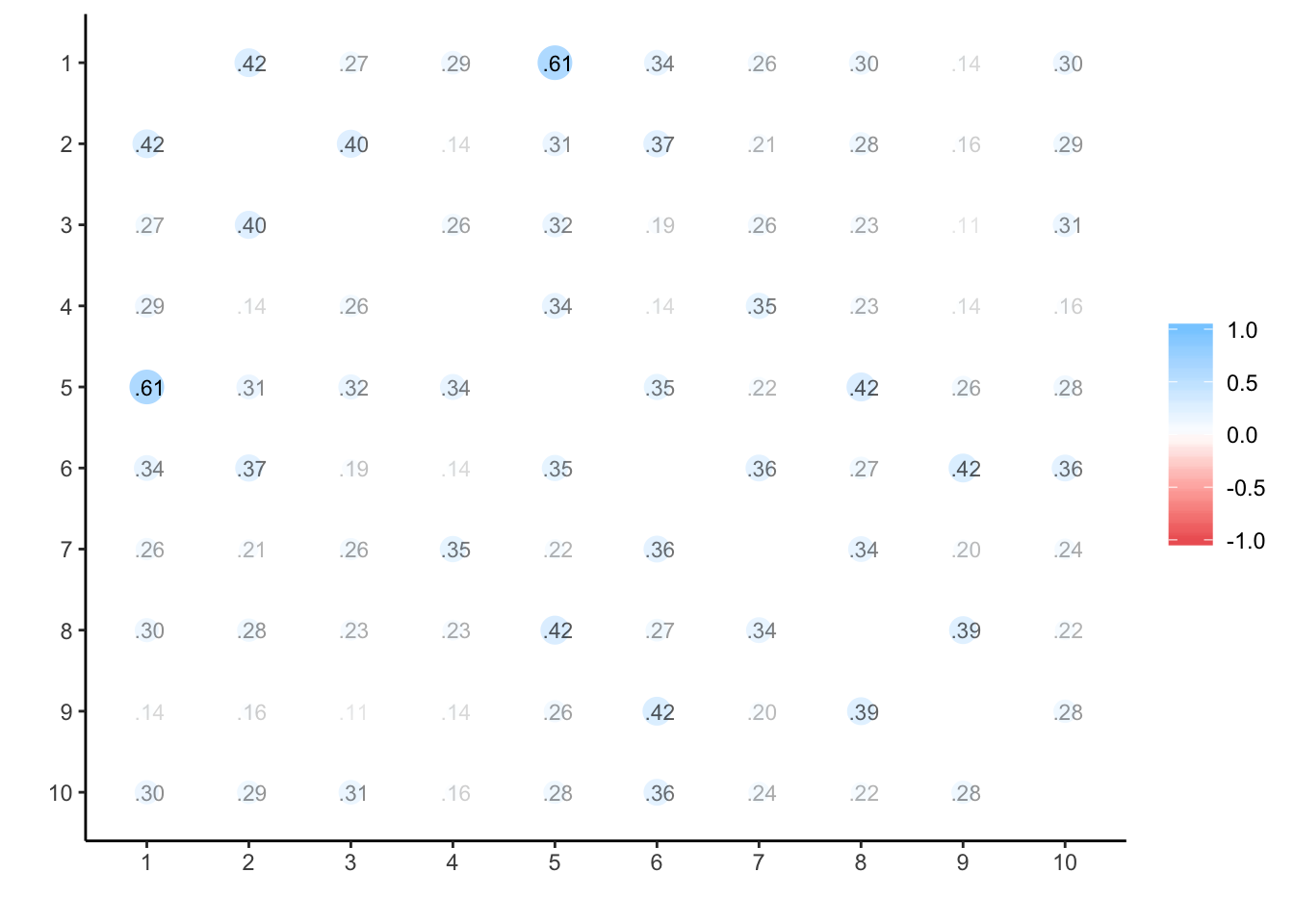### Testosterone

multilevel.reliability(data_wide %>% select(oc_id, date, test), "oc_id", "date", 3, aov = F)
## Warning in cov2cor(C): diag(.) had 0 or NA entries; non-finite result is
## doubtful
##
## Multilevel Generalizability analysis
## Call: multilevel.reliability(x = data_wide %>% select(oc_id, date,
##     test), grp = "oc_id", Time = "date", items = 3, aov = F)
##
## The data had  352  observations taken over  306  time intervals for  1 items.
##
##  Alternative estimates of reliability based upon Generalizability theory
##
## RkRn =  1 Generalizability of between person differences averaged over time (time nested within people)
## Rcn  =  0.83 Generalizability of within person variations averaged over items  (time nested within people)
##  The nested components of variance estimated from lme are:
##          Variance Percent
## id            432   0.573
## id(time)      268   0.355
## residual       55   0.072
## total         755   1.000
##
## To see the ANOVA and alpha by subject, use the short = FALSE option.
##  To see the summaries of the ICCs by subject and time, use all=TRUE
##  To see specific objects select from the following list:
##  ANOVA s.lmer s.lme alpha summary.by.person summary.by.time ICC.by.person ICC.by.time lmer long Call
data_wide %>%
select(oc_id, timepoint, test) %>%
select(-oc_id) %>%
cor(use='na.or.complete') %>%
corrr::rplot(print_cor = T)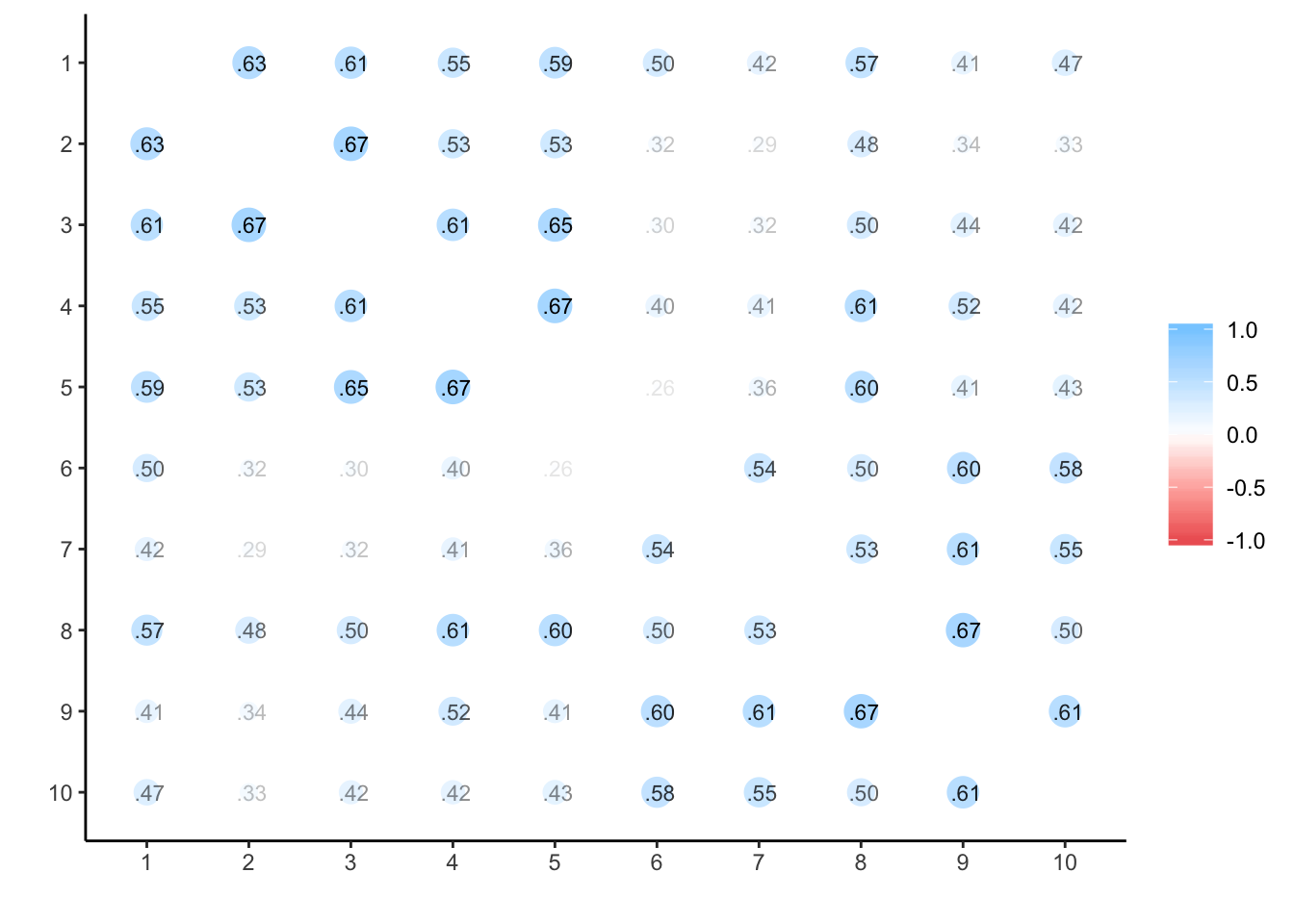# data_wide %>% select(oc_id, date, test) %>% group_by(oc_id) %>%
#   arrange(oc_id, date) %>%
#   mutate(
#     date = as.Date(date),
#     time_since_start = round(as.numeric(date - min(date)))) %>%
#   ungroup() %>%
#   select(-oc_id, -date) %>%
#   cor(use='na.or.complete') %>%
#   corrr::rplot(print_cor = T)

### Cortisol

multilevel.reliability(data_wide %>% select(oc_id, date, cort), "oc_id", "date", 3, aov = F)
## Warning in cov2cor(C): diag(.) had 0 or NA entries; non-finite result is
## doubtful

## Warning in cov2cor(C): diag(.) had 0 or NA entries; non-finite result is
## doubtful
##
## Multilevel Generalizability analysis
## Call: multilevel.reliability(x = data_wide %>% select(oc_id, date,
##     cort), grp = "oc_id", Time = "date", items = 3, aov = F)
##
## The data had  352  observations taken over  306  time intervals for  1 items.
##
##  Alternative estimates of reliability based upon Generalizability theory
##
## RkRn =  1 Generalizability of between person differences averaged over time (time nested within people)
## Rcn  =  0.85 Generalizability of within person variations averaged over items  (time nested within people)
##  The nested components of variance estimated from lme are:
##          Variance Percent
## id         0.0123   0.432
## id(time)   0.0137   0.481
## residual   0.0025   0.087
## total      0.0285   1.000
##
## To see the ANOVA and alpha by subject, use the short = FALSE option.
##  To see the summaries of the ICCs by subject and time, use all=TRUE
##  To see specific objects select from the following list:
##  ANOVA s.lmer s.lme alpha summary.by.person summary.by.time ICC.by.person ICC.by.time lmer long Call
data_wide %>%
select(oc_id, timepoint, cort) %>%
corrr::rplot(print_cor = T)